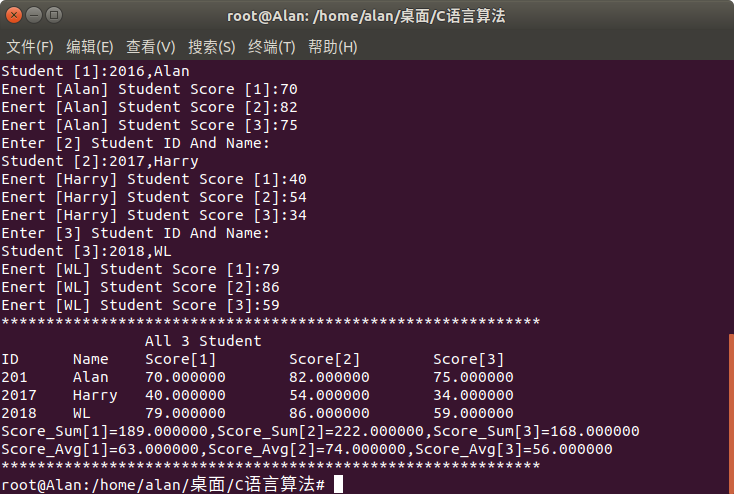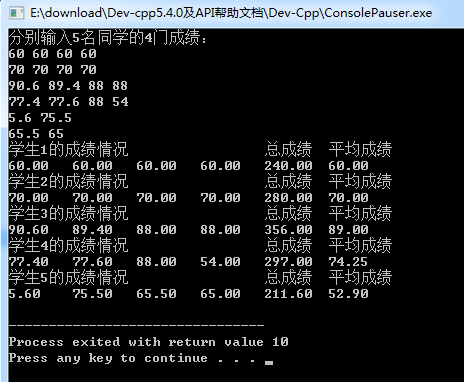• 输入n个学生的学号、姓名、数学成绩、语文成绩和英语成绩，位学生的三门课程的总分，然后按总分由高到低排序，并将排序结果按学号、姓名、数学成绩、语文成绩、英语成绩、总分和名次打印输出到屏幕和文件 ...
Question:
输入n个学生的学号、姓名、数学成绩、语文成绩和英语成绩，求出每位学生的三门课程的总分，然后按总分由高到低排序，并将排序结果按学号、姓名、数学成绩、语文成绩、英语成绩、总分和名次打印输出到屏幕和文件 student.dat中。要求用结构体描述学生信息，输出打印时每位学生信息占一行且上下列对齐，总分相同时名次相同，例如，假设有四个成绩为280、275、275、250，则对应的名次为1、2、2、4。
Code:
#include<stdio.h>
#include<string.h>
#include<stdlib.h>

struct student
{
int id;			// 学号
char name;	// 姓名
int math;		// 数学成绩
int chinese;	// 语文成绩
int English;	// 英语成绩
}a;

// 输入学生的相关信息
void input(){
for(int i=0; i<4; i++){
scanf("%d %s %d %d %d", &a[i].id, a[i].name, &a[i].math, &a[i].chinese, &a[i].English);
}
}

// 对学生的成绩进行排排名
void sort(){
for(int i=0; i<4; i++){
for(int j=i; j<4; j++){
if((a[i].math+a[i].chinese+a[i].English) < (a[j].math+a[j].chinese+a[j].English)){
a.id=a[j].id; strcpy(a.name, a[j].name); a.math=a[j].math; a.chinese=a[j].chinese; a.English=a[j].English;
a[j].id=a[i].id; strcpy(a[j].name, a[i].name); a[j].math=a[i].math; a[j].chinese=a[i].chinese; a[j].English=a[i].English;
a[i].id=a.id; strcpy(a[i].name, a.name); a[i].math=a.math; a[i].chinese=a.chinese; a[i].English=a.English;
}
}
}
}

// 将程序进行输入并写入到文件中
int print(){
int rank;	// 排名
FILE *fp;	// 定义文件指针
fp = fopen("student.dat", "w");		// 将文件指针指向文件

// 判断磁盘中的文件是否存在
if(fp == NULL)
{
printf("磁盘中文件不存在!");
exit(0);
}
fprintf(fp, "学号: %d 姓名: %s 数学成绩: %d 语文成绩: %d 英语成绩: %d 总分: %d 名次: 1\n", a.id, a.name, a.math, a.chinese, a.English, a.math+a.chinese+a.English);

for(int i=1; i<4; i++){
rank=i+1;
if((a[i].math+a[i].chinese+a[i].English) == (a[i-1].math+a[i-1].chinese+a[i-1].English)){
rank=i;
}
fprintf(fp, "学号: %d 姓名: %s 数学成绩: %d 语文成绩: %d 英语成绩: %d 总分: %d 名次: %d\n", a[i].id, a[i].name, a[i].math, a[i].chinese, a[i].English, a[i].math+a[i].chinese+a[i].English, rank);
}
fclose(fp);

printf("\n\n");
printf("学号: %d 姓名: %s 数学成绩: %d 语文成绩: %d 英语成绩: %d 总分: %d 名次: 1\n", a.id, a.name, a.math, a.chinese, a.English, a.math+a.chinese+a.English);
for(int i=1; i<4; i++){
rank=i+1;
if((a[i].math+a[i].chinese+a[i].English) == (a[i-1].math+a[i-1].chinese+a[i-1].English)){
rank=i;
}
printf("学号: %d 姓名: %s 数学成绩: %d 语文成绩: %d 英语成绩: %d 总分: %d 名次: %d\n", a[i].id, a[i].name, a[i].math, a[i].chinese, a[i].English, a[i].math+a[i].chinese+a[i].English, rank);
}
}

int main(){
int i, j;			// 两个for循环用到的i, j, 定义的排名变量rank

printf("请输入学生的相关信息:\n");
input();	// 信息输入
sort();		// 成绩排名
print();	// 将成绩信息打印到屏幕和文件
return 0;
}


测试数据:
100 张三 99 98 97
101 李四 89 88 87
102 王五 79 78 77
103 赵六 99 98 97

Result:Get:
1、c语言的写入文件的方式(定义指针-> 指向文件 -> 判断文件存在 -> fprintf)
2、熟悉结构体定义以及调用的方式(scanf输入用地址，否则用实体)


展开全文结构体 写入文件 文件指针
• 要求设计一程序:输入学生的学号、姓名、和三门成绩，最后输出三门成绩的总分和平均分 设计代码: //程序功能: 假设一班有30名学生 试写一程序 存放这30名学生的学号、姓名、三门课程的成绩 最后根据30名...
【C语言】假设一个班30名学生。要求设计一个程序:输入每位学生的学号、姓名、和三门成绩，最后输出三门成绩的总分和平均分

设计代码:

//程序功能: 假设一个班有30名学生 试写一个程序 存放这30名学生的学号、姓名、三门课程的成绩  最后根据30名同学的成绩求出每门课的平均分；

#include "stdio.h"

#define N 3 //设置常量控制学生数

//建立结构体
struct Student {

int Id;//存放学生的学号
char Name;//存放学生姓名
float Score;//存放三门课的成绩
} stu[N];

int main() {

int i,j;
float Sum = {0},Avg = {1};
//首先输入:
for(i = 0;i < N;i++) {

printf("Enter [%d] Student ID And Name:\nStudent [%d]:",i+1,i+1);
scanf("%d,%s",&stu[i].Id,stu[i].Name);
for(j = 0;j < 3;j++) {

printf("Enert [%s] Student Score [%d]:",stu[i].Name,j+1);
scanf("%f",&stu[i].Score[j]);

}
}

//全部信息输出
for(i = 0;i < 60;i ++){
printf("*");
}
printf("\n\t\tAll %d Student\n",N);
printf("ID\tName\tScore\tScore\tScore\t\n");
for(i = 0; i < N;i++) {
printf("%d\t%s\t%f\t%f\t%f\n",stu[i].Id,stu[i].Name,stu[i].Score,stu[i].Score,stu[i].Score);
}

//计算学科平均分和总和
for(i = 0;i < N;i++) {

Sum += stu[i].Score;
Sum += stu[i].Score;
Sum += stu[i].Score;
Avg = Sum / 3;
Avg = Sum / 3;
Avg = Sum / 3;
}

//输出每门成绩的信息
printf("Score_Sum=%f,Score_Sum=%f,Score_Sum=%f\nScore_Avg=%f,Score_Avg=%f,Score_Avg=%f\n",Sum,Sum,Sum,Avg,Avg,Avg);

for(i = 0;i < 60;i++) {
printf("*");
}
printf("\n");

return 0;
}

运行结果:大家也可以关注我的个人网站一起来进行讨论

展开全文• 《程序设计基础实训指导...【实训内容5】有5个学生，每个学生修4门课程，求每个学生所有成绩的总分和平均分 #include <stdio.h> #define First 5 #define Second 6 int main(void) { float a[First][Second],...
《程序设计基础实训指导教程-c语言》
ISBN 978-7-03-032846-5
p73
4.1.2 上级实训内容

【实训内容5】有5个学生，每个学生修4门课程，求每个学生所有成绩的总分和平均分
#include <stdio.h>
#define First  5
#define Second 6
int main(void)
{
float a[First][Second],sum=0.0;
int i,j,cnt=1;
printf("分别输入5名同学的4门成绩：\n");
for(i=0;i<First;i++)
{
for(j=0;j<Second-2;j++)
{
scanf("%f",&a[i][j]);
}
}
for(i=0;i<First;i++)
{
for(j=0;j<Second-2;j++)
{
sum+=a[i][j];
}
a[i]=sum;
a[i]=a[i]/4.0;
sum=0;	//初始化
}
for(i=0;i<First;cnt++,i++)
{
printf("学生%d的成绩情况\t\t\t总成绩\t平均成绩\n",cnt);
for(j=0;j<Second;j++)
{
printf("%.2f\t",a[i][j]);
}
printf("\n");
}
}展开全文• 每个学生的信息为：学号、姓名、性别、家庭住址、联系电话、语文、数学、外语三门单科成绩、考试平均成绩、考试名次、同学互评分、品德成绩、任课教师评分、综合测评总分、综合测评名次。考试平均成绩、同学互评分、...c++
• 用函数传参出表内，个个学生总分和平均分，以及每个科目分别的总分平均分c
• 有5名学生，每个学生的数据包括学号、姓名、英语成绩、数学成绩、C语言成绩，从键盘输入5名学生的数据，要求输出5名学生的总平均成绩，以及总分最高的学生的数据（包括学号、姓名、3门课程的成绩、总分数）。
• 学生量化总分降序排序（10一行），并输出低于平均分的量化总分（假设有20名学生）跪大佬！！！</p>有问必答 c++
• finput()输入每个学生信息 单科和总分最高的学生信息 代码展示1. #define _CRT_SECURE_NO_WARNINGS #include<iostream> #include<stdio.h> #include <string.h> //void ...
问题简介：

学生信息：姓名，学号，英语成绩，数学成绩，政治成绩

写出学生结构体信息
finput()输入每个学生信息
求单科和总分最高的学生信息
代码展示1.

#define _CRT_SECURE_NO_WARNINGS
#include<iostream>
#include<stdio.h>
#include <string.h>
//void finput();
struct student{
char name;
int ID;
int English;
int 	Math;
int political;
};
student finput(struct student std){
//struct student std;
printf("请输入学生的姓名，学号，成绩(英语，数学，政治):\n");
printf("姓名:");
scanf("%s", std.name);
printf("学号:");
scanf("%d", std.ID);
printf("英语:");
scanf("%d", &std.English);
printf("数学:");
scanf("%d", &std.Math);
printf("政治:");
scanf("%d", &std.political);
return std;
}
int maxScore(int a[]){
int j;
int max = 0;
for (int i = 0; i < 3; i++){
if (a[i]>max){
max = a[i];
j = i;
}
}
return j;
}
int compare(int x1, int x2, int x3){
int max = 0;
if (x1 > x2){
max = x1;
}
else{
max = x2;
}
if (x3 > max){
max = x3;
}
return max;
}
int main(){
struct student std;
for (int i = 0; i < 3; i++){
std[i] = finput(std[i]);
printf("\n");
}
int sum = {0,0,0};
int math = {0,0,0};
int english = {0,0,0};
int political;
for (int i = 0; i < 3; i++){
sum[i] = std[i].English + std[i].Math + std[i].political;
printf("%d \t %d \t %d \n",std[i].English, std[i].Math, std[i].political);
printf("%d\n", sum[i]);
math[i] = std[i].Math;
english[i] = std[i].English;
political[i] = std[i].political;
}
int index = maxScore(sum);
int index1 = maxScore(math);
int index2 = maxScore(english);
int index3 = maxScore(political);
printf("总分成绩最高的学生成绩%d,学生名为%s\n", sum[index], std[index].name);
//英语
printf("英语成绩最高的学生成绩%d,学生名为%s\n", english[index2], std[index2].name);
//数学
printf("数学成绩最高的学生成绩%d,学生名为%s\n", math[index1], std[index1].name);
//政治
printf("政治成绩最高的学生成绩%d,学生名为%s\n", political[index3], std[index3].name);

}

代码展示2.

//有n个结构体变量，内含学生号， 姓名和三门课成绩。要求输出单科成绩和总成绩最高学生的信息。
#include <stdio.h>
#define N 3
struct Student
{
int num;
char name;
float socre;
float total_points;
};
void input(struct Student stu[])
{
int i;
printf("请分别输入各学生的信息：学号，姓名，三门课成绩\n");
for(i=0;i<N;i++)
//score是英语成绩， score是数学成绩成绩，score是政治成绩
{
scanf("%d %s %f %f %f",&stu[i].num,stu[i].name,&stu[i].socre,&stu[i].socre,&stu[i].socre);
stu[i].total_points=(stu[i].socre+stu[i].socre+stu[i].socre);
}
}
struct Student max(struct Student stu[])
{
int i,m=0;
for(i=0;i<N;i++)
if(stu[i].total_points>stu[m].total_points)
m=i;
return stu[m];
}
struct Student english_max(struct Student stu[])
{
int i,m=0;
for(i=0;i<N;i++){
if(stu[i].socre>stu[m].socre){
m=i;
}
}
return stu[m];
}
struct Student math_max(struct Student stu[])
{
int i,m=0;
for(i=0;i<N;i++){
if(stu[i].socre>stu[m].socre){
m=i;
}
}
return stu[m];
}
struct Student political_max(struct Student stu[])
{
int i,m=0;
for(i=0;i<N;i++){
if(stu[i].socre>stu[m].socre){
m=i;
}
}
return stu[m];
}
void print1(struct Student stud)
{
printf("单科英语成绩最高的学生是：\n");
printf("学号：%d\n姓名:%s\n英语成绩：%6.2f\n",stud.num,stud.name,stud.socre);
//printf("单科数学成绩最高的学生是：\n");
//printf("学号：%d\n姓名:%s\n英语成绩：%6.2f\n",stud.num,stud.name,stud.socre);
//printf("单科政治成绩最高的学生是：\n");
//printf("学号：%d\n姓名:%s\n英语成绩：%6.2f\n",stud.num,stud.name,stud.socre);

}
void print2(struct Student stud)
{
printf("单科数学成绩最高的学生是：\n");
printf("学号：%d\n姓名:%s\n英语成绩：%6.2f\n",stud.num,stud.name,stud.socre);

}
void print3(struct Student stud)
{
printf("单科政治成绩最高的学生是：\n");
printf("学号：%d\n姓名:%s\n英语成绩：%6.2f\n",stud.num,stud.name,stud.socre);

}
void print(struct Student stud)
{

printf("成绩最高的学生是：\n");
printf("学号：%d\n姓名：%s\n三门课成绩：%5.1f,%5.1f,%5.1f\n总成绩：%6.2f\n",stud.num,stud.name,stud.socre,stud.socre,stud.socre,stud.total_points);
}

int main()
{
struct Student stu[N],*p=stu;
input(p);
print1(english_max(p));
print2(math_max(p));
print3(political_max(p));
print(max(p));
return 0;
}

第二种方法可改进。留给查看的博友吧！

展开全文• 设学生成绩已以一个学生一个记录的形式存储在文件中， 位学生记录包含的信息有：姓名，学号和各门功课的成绩。 程序具有以下几项功能：出各门课程的总分，平均分，按姓名， 按学号寻找其记录并显示，浏览全部...
• （1）计算每个学生总分和平均分； （2）按总分成绩由高到低排出成绩的名次； （3）打印出名次表，表格内包括学生编号、各科分数、总分和平均分； （4）任意输入一个学号，能够查找出该学生在班级中的排名及其考试...
• 功能： 录入成绩 找三科总分的最高分 最低分 算出平均分场景头文件成绩结构体合班级结构体头插法创建成绩创建班级求总分最大求总分最小求总分平均分主函数 场景：一个年级，相当链表A 该年级5个班，每个班5个人，...链表
• 用结构体存放每个学生的信息，包括学号、姓名、3门课程的成绩、总分、平均分和排名。（2）能通过键盘录入学生信息，包括学号、姓名和3门课程的成绩。（3）能计算每名学生的总分...有问必答 c++
• 定义一个学生成绩的结构体数组，其中的数据成员包括学号（char num）、姓名(name)、三门课的成绩（grade）、总分（score）、均分（average））。 （1）从键盘上输入N名学生的信息（N定义为常变量）； （2）...
• 设计某班学生成绩管理系统，要求实现以下功能： 1、从键盘输入学号、姓名、各门课程成绩（不少于...2、打开文件后，计算每个人的总分和平均分，排序并保存。 3、可以在文件中进行单项查询或多项查询的功能。 万谢c 代码
• 选择界面②用二维数组和调用函数实现学号、语文、数 学、英语成绩的输入、求每-一人的总成绩、平均分、显 示、查询、修改和排序(按总分)功能、输出各科最高分、 以及排序后的成绩表(包含表头)。
• 6求每个学生的平均分，总分及所有学生的总分，各科成绩的平均分。 8按学号，成绩进行排序。 9对已作过的修改，及输入的数据进行存盘。下次启动总是最新数据。? 本程序的一大特点：是可以对具有相同学号，姓名，系的...
• 统计3个班成绩的情况,每个班有5名同学,每个班的平均分和所以班级的平均分(学生的成绩从键盘输入) 思路分析 分解题目,先处理简单的,再处理复杂的,根据需求增加或修改相应的代码 各个班的平均分 所有班级的...
• 定义一个学生成绩的结构体数组，其中的数据成员包括学号（char num）、姓名(name)、三门课的成绩（grade）、总分（score）、均分（average））。 （1）从键盘上输入N名学生的信息（N定义为常变量）； （2）...
• （2）编写一个程序实现如下功能：输入10个学生5门课程的成绩，分别用函数：①每个学生的平均分；②每门课程的平均分；③找出最高的分数所对应的学生和课程。 若输入2个学生的成绩，其运行结果如下图所示。 （3...
• 一丶PTA实验作业 题目1：结构体数组按总分排序 （1）.PTA提交列表 ...for i=0 to n-1 //每个学生分数的总和 for j=0 to 2 执行算法（p+i）->sum=(p+i)->sum+(p+i)->score[j] end f...数据结构与算法
• 学生总分 定义i,j循环变量 for i=0 to n for j=0 to 3 p[i].sum+=p[i].score[j]将三成绩累加即为总分 end 总分从高到低排序 定义i,j循环变量 定义结构体中间变量t for i=0 to 5 for j=0 to...
• 成员表由若干个成员组成， 每个成员都是该结构的一个组成部分。对每个成员也必须作类型说明，其形式为： 类型说明符 成员名; 成员名的命名应符合标识符的书写规定。例如： struct stu { int num; char name; ...
• 全班47个人，7门课的成绩 （1） 从键盘上输入学生姓名和成绩。...全班每个人七门课的总分和平均分。并找出最高分和最低分的人。 ( 3 )全班每个人七门课的总分和平均分。并找出最高分和最低分的人12
• 输入5个学生的数学、英语、物理和计算机4门课的成绩，调用函数计算每个学生总分，返回最高总分和最低总分。 提示：在主函数中输入数据、显示最高总分和最低总分 我的代码 #include #include int max = 0, min...c float 二维数组 函数
• 每个学生的信息为：学号、姓名、性别、课程单科成绩（包括C语言、高数、大学英语、总分）、总分、平均成绩。 主要功能： (1)按学号顺序输入学生信息，包括学号、姓名、班级、性别、课程单科成绩（包括C语言、高数...c#
• 4.N个学生M门课，所有学生的各门课程成绩已存入文件f1.dat，请计算每个学生总分，并按总分降序的次序将每个学生名次、各门课程得分及总分输出到文件f2.dat。要求：在按总分求学生名次时，不得对学生原始数据排序。...c 冒泡排序 单链表
• 班级有50名学生学生选修301 ]课程。要求: 1.设计算法，出该班级门课程的平均分...2.学生各门课程的合计总分，按照总分由高到低排序。给出求解上述问题算法的传统流程图和相应的C语言程序。 ...
• [正文]1 需求分析 在现在的许多大学或者高中小学生活学习中，体育锻炼越来越受到学校和国家的关注，而在学生当中，更是受到了大家的喜爱，于是每个学校都会有自己的体育运动会，各种各样的项目比赛，激烈非常。...
• 对一个有N个学生的班级，每个学生有M门课程。该系统实现对班级成绩的录入、显示、修改、排序、保存等操作的管理。 二、功能要求： 1、本系统采用一个结构体数组，每个数据的结构应当包括：学号、姓名、M门课程名称...
• 高斯在上小学的一天，老师要求每个学生都计算1+2+…+100的结果，谁先算出来谁先回家…… 2.4算法定义 20 现实世界中的算法千变万化，没有通用算法可以解决所有问题。甚至一个小问题，某个解决此类问题很优秀的算法...数据结构# c语言求每个学生总分c语言 订阅